# IB DP Physics: SL复习笔记5.1.4 Electric Fields

### Electric Fields

• An electric field is a region of space in which an electric charge “feels” a force
• It is a vector field
• The direction of the electric field is the same as the direction of the electric force

#### Electric Field Strength

• The electric field strength is a measure of the strength of the electric field

The electric field strength is defined as the magnitude of the electric force per unit charge experienced by a small positive test charge placed at that point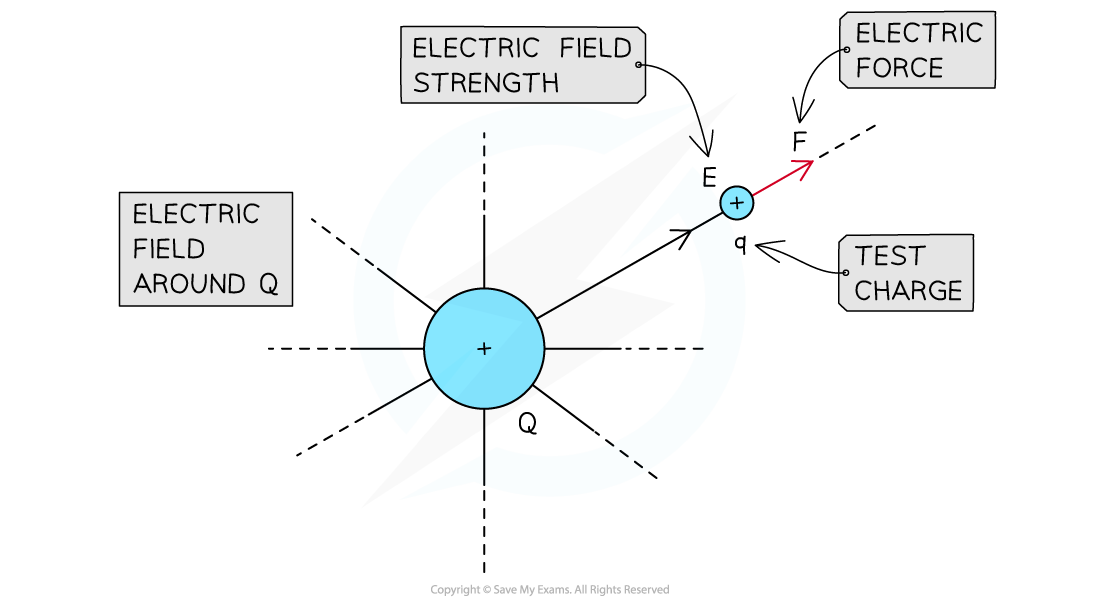The strength of the electric field generated by charge Q is measured by placing a test charge q in the field

• The equation to calculate the electric field strength at a given point in space is: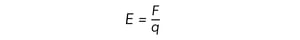• Where:
• E = electric field strength in newtons per coulomb N C–1
• F = electric force in newtons (N)
• q = electric charge in coulombs (C)

#### Representing Electric Fields

• Electric field lines are used to visualise electric fields
• Arrows along the lines indicate the direction of the field
• The arrows always point away from the positive charge and towards negative the charge
• Around a point charge or a charged sphere, the electric field lines are directly radially inwards or outwards:
• If the charge is positive (+), the field lines are radially outwards
• If the charge is negative (–), the field lines are radially inwards
• This shares many similarities to radial gravitational field lines around a point mass
• The difference being that the gravitational force is always attractive, whilst the electric force can be either attractive or repulsiveElectric field lines around isolated positive and negative point charges

• The strength of the electric field is proportional to the number of lines per unit cross-sectional area
• A stronger electric field is represented by arrows that are closer together
• The field lines between opposite charges are connected, showing attraction
• The field lines between like repelling charges never connect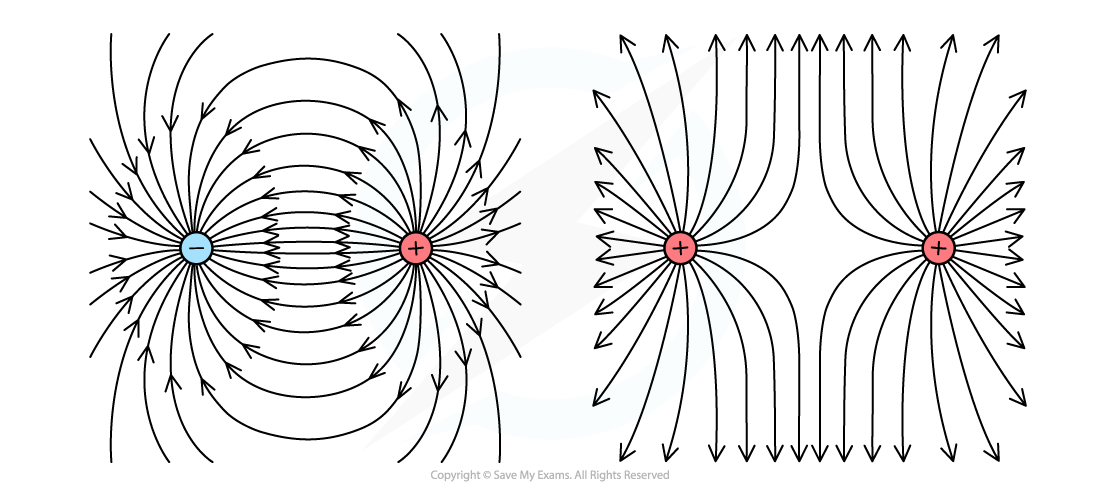Electric field lines showing attraction between a positive and a negative charge, and repulsion between two positive charges

#### Uniform and Non-Uniform Electric Fields

• A uniform electric field is a field of force in which the strength of the electric force is the same throughout
• It is represented by parallel and equally spaced field lines
• Whenever the spacing between the field lines changes, the electric field is non-uniform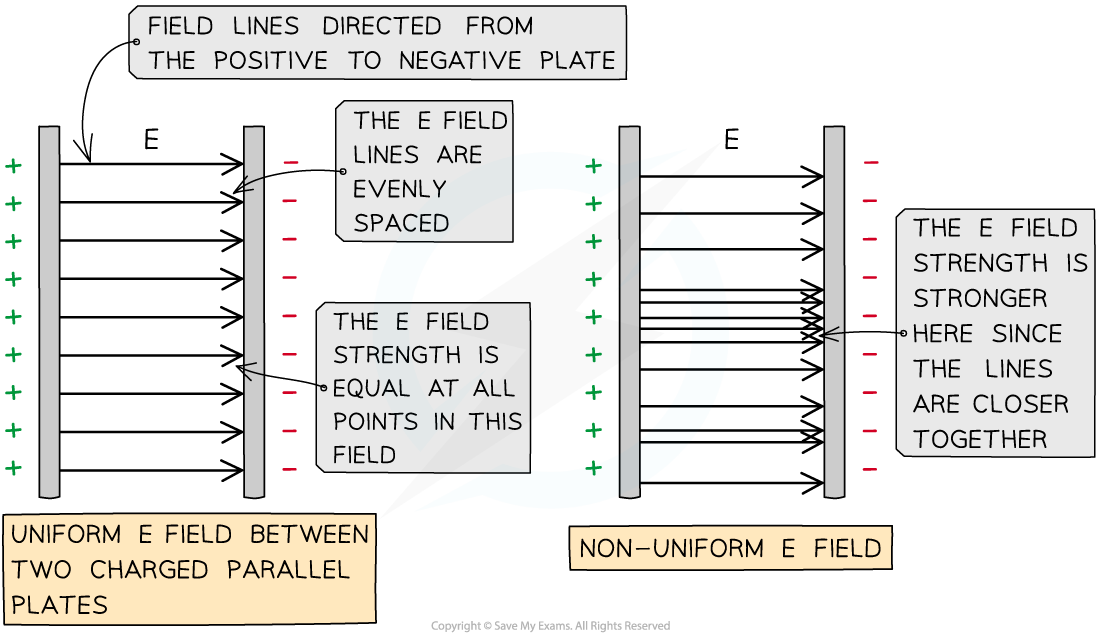Uniform and non-uniform electric fields between two parallel plates

#### Worked Example

A charged particle is in an electric field with electric field strength 3.5 × 104 N C-1, where it experiences a force of 0.3 N.Calculate the charge of the particle.

Step 1: Write down the known quantities

• E = 3.5 × 104 N C-1
• F = 0.3 N

Step 2: Write down the equation for the electric field strengthStep 3: Rearrange the above equation to calculate the charge q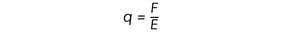Step 4: Substitute the numbers into the above equation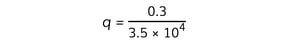q = 8.6 × 10–6 C

#### Exam Tip

When drawing field lines, make sure they never cross. The electric field can only have one value at any given point.